Cellular Principles Cellular Hierarchy Cellular Principles 2 System

• Slides: 83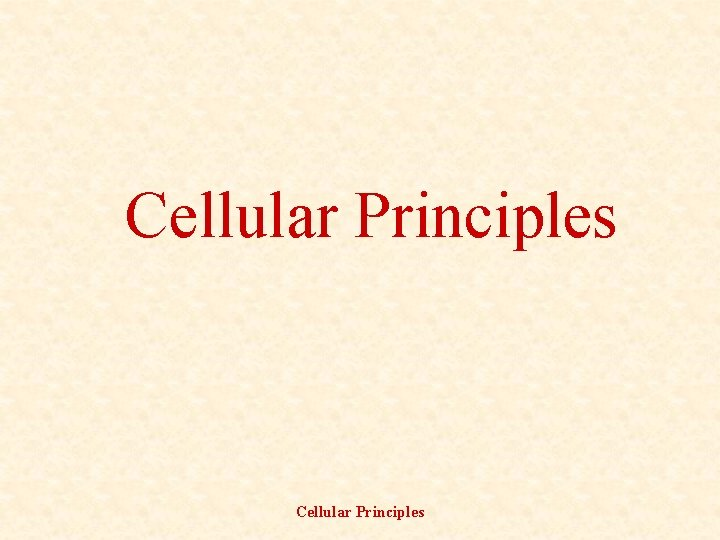Cellular Principles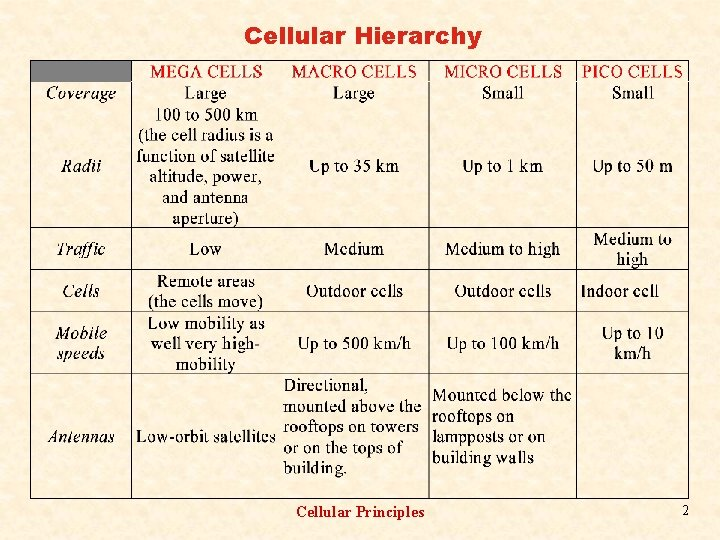Cellular Hierarchy Cellular Principles 2System Management q Link Quality Measurement q Forward and reverse links are continually monitored q Parameters: received signal quality and the bit error rates q Cell Selection q Choice of operator q User preferences q Available Networks q MS capabilities q Network capabilities q MS mobility q Service requirements Cellular Principles 3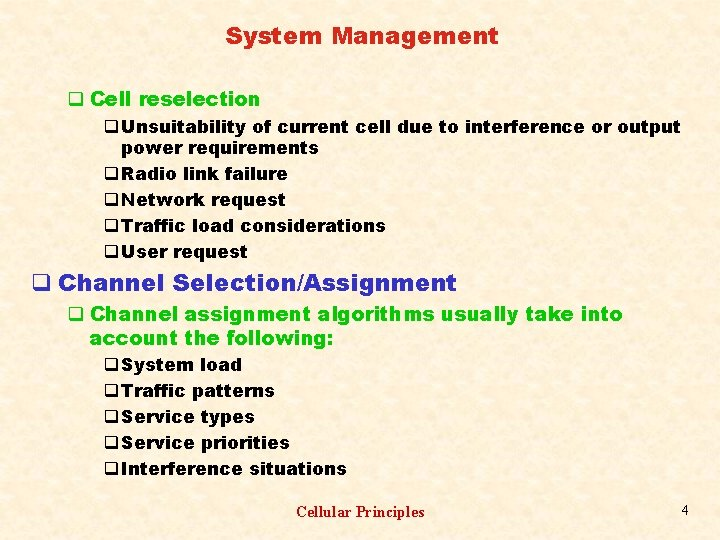System Management q Cell reselection q Unsuitability of current cell due to interference or output power requirements q Radio link failure q Network request q Traffic load considerations q User request q Channel Selection/Assignment q Channel assignment algorithms usually take into account the following: q System load q Traffic patterns q Service types q Service priorities q Interference situations Cellular Principles 4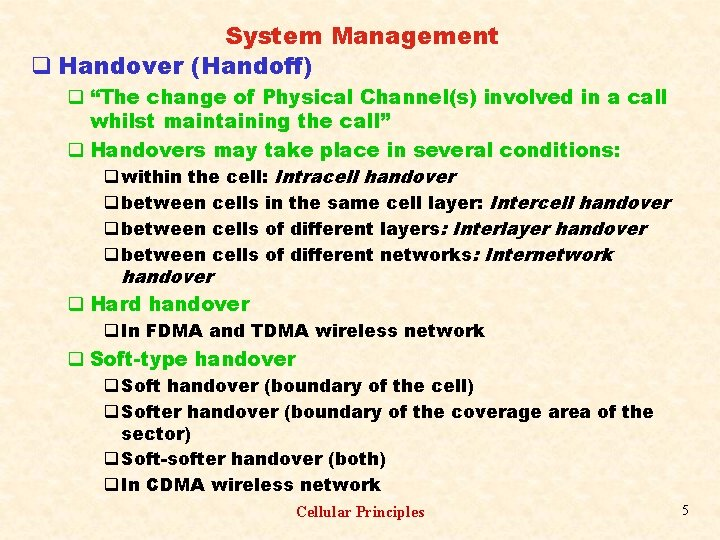System Management q Handover (Handoff) q “The change of Physical Channel(s) involved in a call whilst maintaining the call” q Handovers may take place in several conditions: q within the cell: Intracell handover q between cells in the same cell layer: Intercell handover q between cells of different layers: Interlayer handover q between cells of different networks: Internetwork handover q Hard handover q In FDMA and TDMA wireless network q Soft-type handover q Soft handover (boundary of the cell) q Softer handover (boundary of the coverage area of the sector) q Soft-softer handover (both) q In CDMA wireless network Cellular Principles 5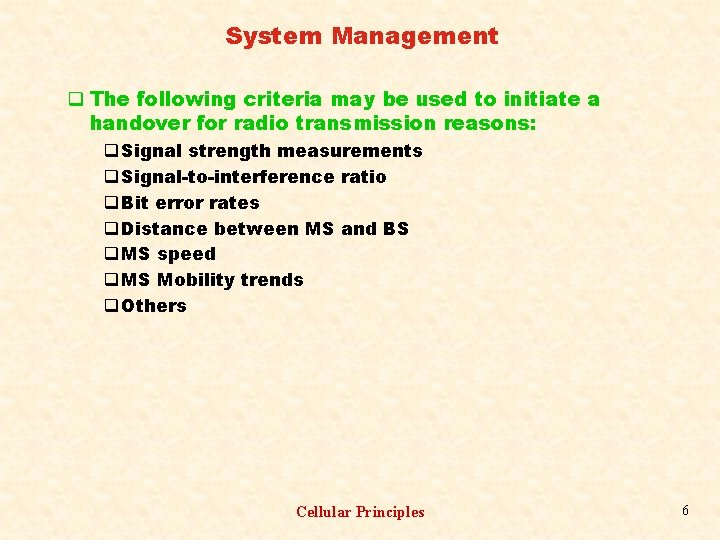System Management q The following criteria may be used to initiate a handover for radio transmission reasons: q Signal strength measurements q Signal-to-interference ratio q Bit error rates q Distance between MS and BS q MS speed q MS Mobility trends q Others Cellular Principles 6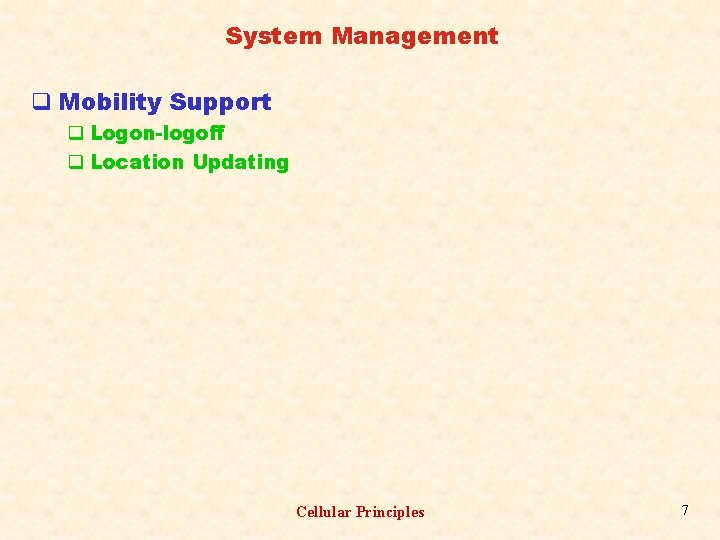System Management q Mobility Support q Logon-logoff q Location Updating Cellular Principles 7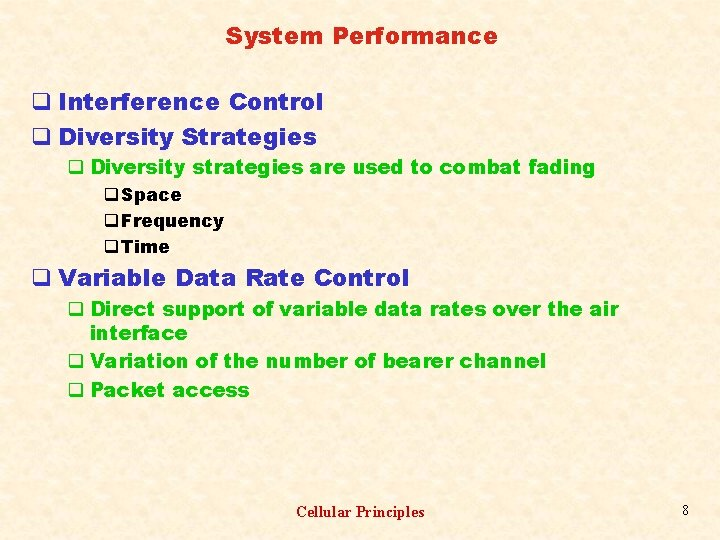System Performance q Interference Control q Diversity Strategies q Diversity strategies are used to combat fading q Space q Frequency q Time q Variable Data Rate Control q Direct support of variable data rates over the air interface q Variation of the number of bearer channel q Packet access Cellular Principles 8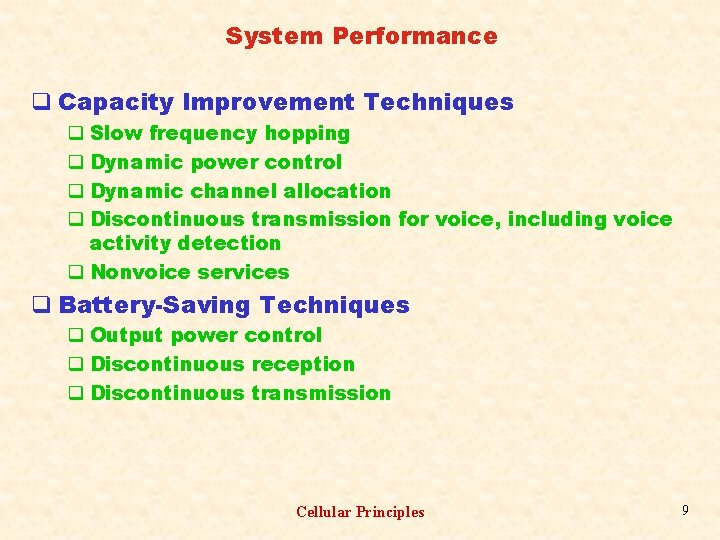System Performance q Capacity Improvement Techniques q Slow frequency hopping q Dynamic power control q Dynamic channel allocation q Discontinuous transmission for voice, including voice activity detection q Nonvoice services q Battery-Saving Techniques q Output power control q Discontinuous reception q Discontinuous transmission Cellular Principles 9Cellular Reuse Pattern q Co-cells: Cells using the same carrier frequency q Cluster: A group of cells among which the whole spectrum is shared and within which no frequency reuse exists q The number of cells per cluster defines the reuse pattern and this is a function of the cellular geometry Cellular Principles 10Macro cellular Reuse Pattern q Circles x Regular Polygons (Equilateral triangles, squares, and hexagons) q Hexagonal cellular geometry q Propagation symmetry q Low-capacity systems Cellular Principles 11Macro cellular Reuse Pattern Cellular Principles 12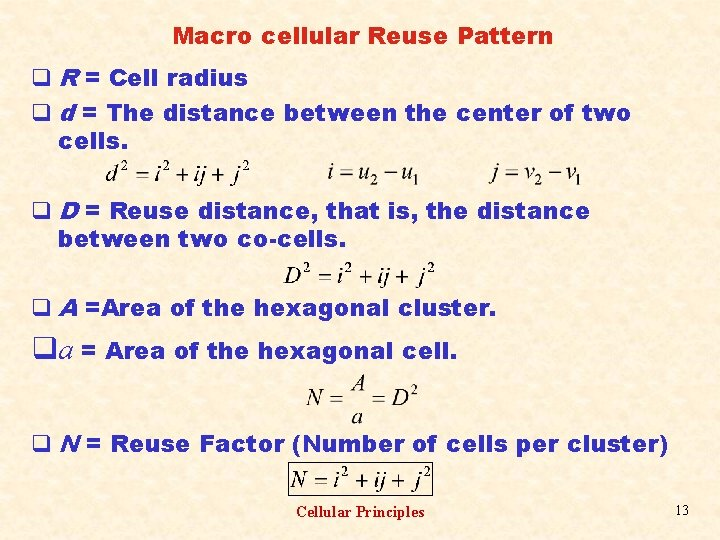Macro cellular Reuse Pattern q R = Cell radius q d = The distance between the center of two cells. q D = Reuse distance, that is, the distance between two co-cells. q A =Area of the hexagonal cluster. qa = Area of the hexagonal cell. q N = Reuse Factor (Number of cells per cluster) Cellular Principles 13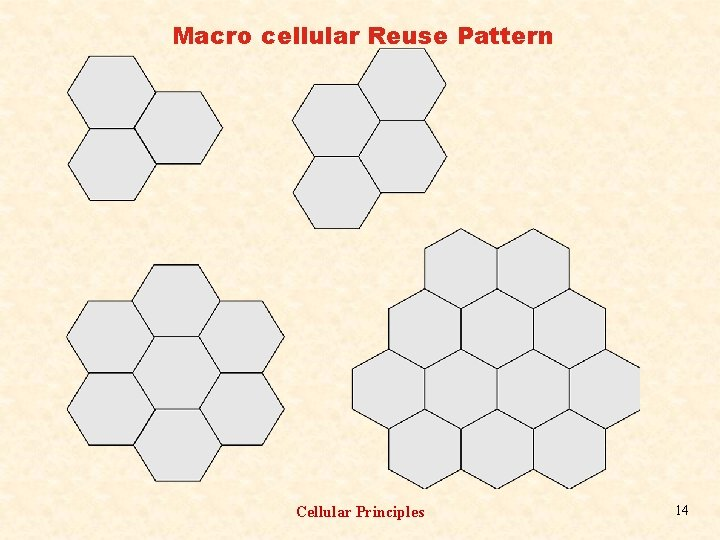Macro cellular Reuse Pattern Cellular Principles 14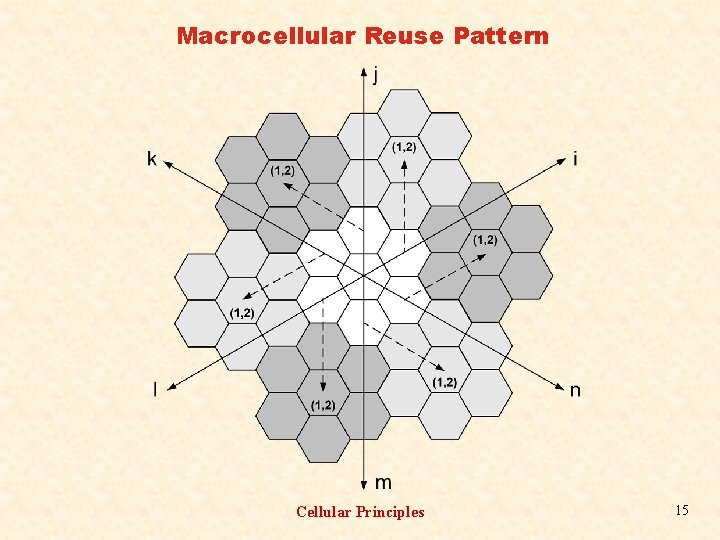Macrocellular Reuse Pattern Cellular Principles 15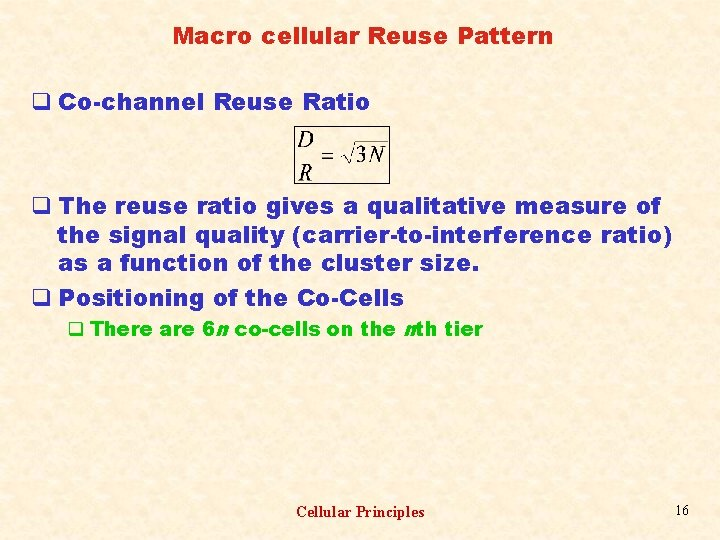Macro cellular Reuse Pattern q Co-channel Reuse Ratio q The reuse ratio gives a qualitative measure of the signal quality (carrier-to-interference ratio) as a function of the cluster size. q Positioning of the Co-Cells q There are 6 n co-cells on the nth tier Cellular Principles 16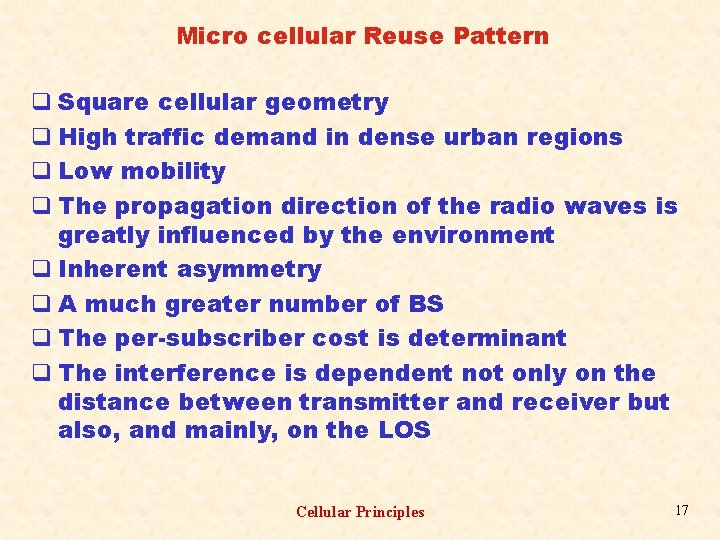Micro cellular Reuse Pattern q Square cellular geometry q High traffic demand in dense urban regions q Low mobility q The propagation direction of the radio waves is greatly influenced by the environment q Inherent asymmetry q A much greater number of BS q The per-subscriber cost is determinant q The interference is dependent not only on the distance between transmitter and receiver but also, and mainly, on the LOS Cellular Principles 17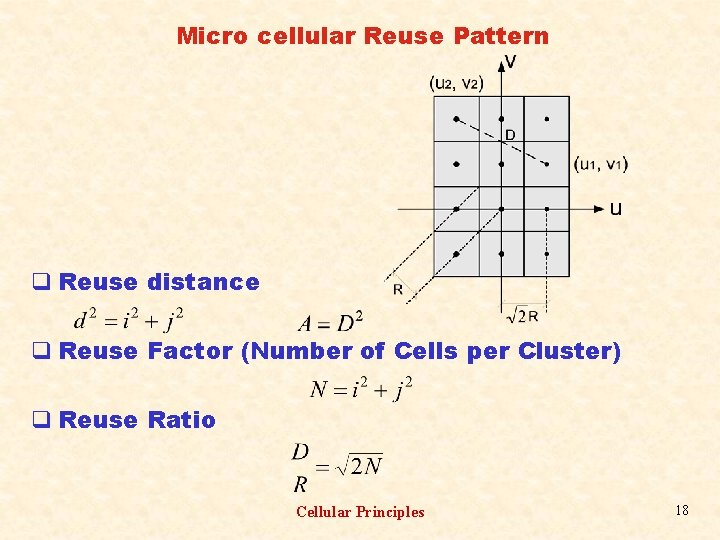Micro cellular Reuse Pattern q Reuse distance q Reuse Factor (Number of Cells per Cluster) q Reuse Ratio Cellular Principles 18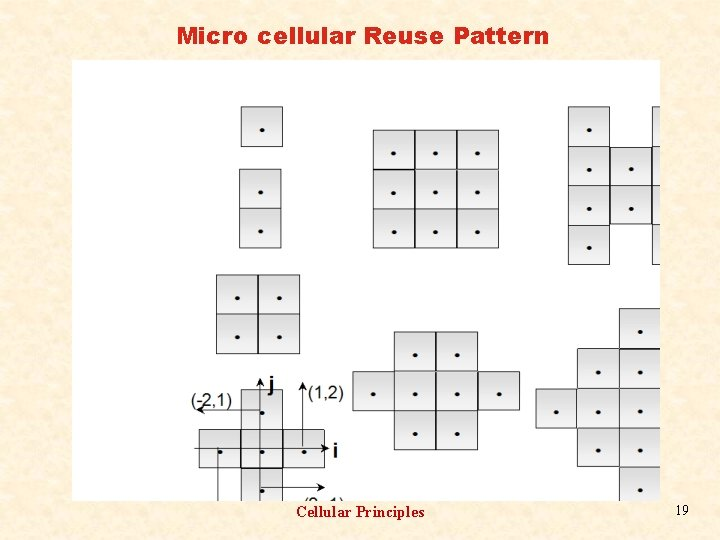Micro cellular Reuse Pattern Cellular Principles 19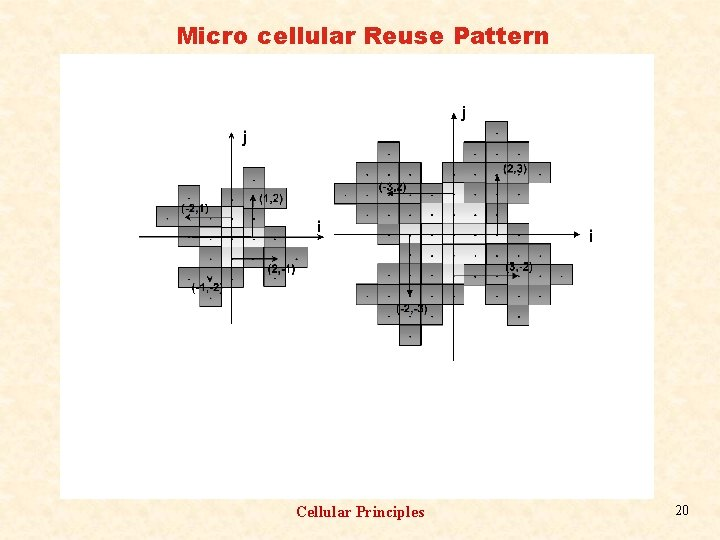Micro cellular Reuse Pattern Cellular Principles 20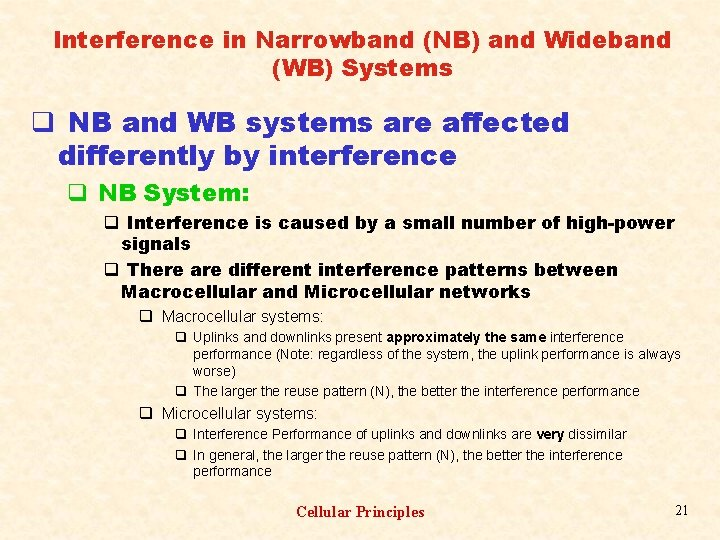Interference in Narrowband (NB) and Wideband (WB) Systems q NB and WB systems are affected differently by interference q NB System: q Interference is caused by a small number of high-power signals q There are different interference patterns between Macrocellular and Microcellular networks q Macrocellular systems: q Uplinks and downlinks present approximately the same interference performance (Note: regardless of the system, the uplink performance is always worse) q The larger the reuse pattern (N), the better the interference performance q Microcellular systems: q Interference Performance of uplinks and downlinks are very dissimilar q In general, the larger the reuse pattern (N), the better the interference performance Cellular Principles 21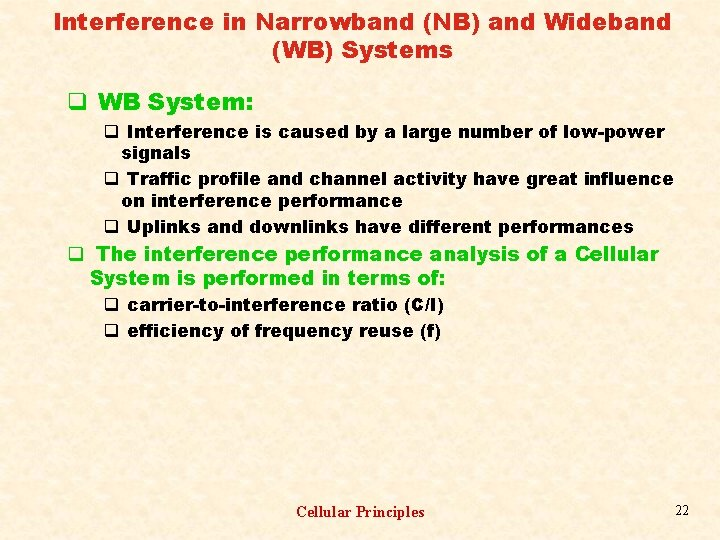Interference in Narrowband (NB) and Wideband (WB) Systems q WB System: q Interference is caused by a large number of low-power signals q Traffic profile and channel activity have great influence on interference performance q Uplinks and downlinks have different performances q The interference performance analysis of a Cellular System is performed in terms of: q carrier-to-interference ratio (C/I) q efficiency of frequency reuse (f) Cellular Principles 22Interference in Narrowband Macrocellular Systems q The propagation is characterized by an NLOS (non line-of-sight) condition q The Mean Power (P) received at a distance (d) from the transmitter is: q K is a proportionality constant that depends on several parameters, such as: f, Base Station (BS) antenna height and gain, Mobile Station (MS) antenna height and gain, environment, etc. q is the propagation path loss coefficient and usually ranges between 2 and 6 Cellular Principles 23Interference in Narrowband Macrocellular Systems q Subsequent calculations assume that: q K and remain constant q MS is positioned for the worst-case condition, that is, at the border of the serving cell (distance R from the BS) q C/I ratio for the downlink is calculated at the MS: q C is the signal power received from the serving BS q I is the sum of the signal powers received from the interfering BS’s (co-cells) Cellular Principles 24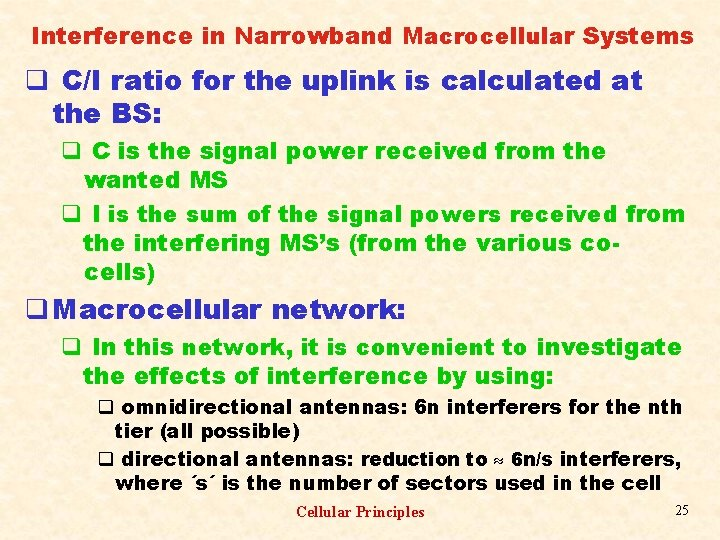Interference in Narrowband Macrocellular Systems q C/I ratio for the uplink is calculated at the BS: q C is the signal power received from the wanted MS q I is the sum of the signal powers received from the interfering MS’s (from the various cocells) q Macrocellular network: q In this network, it is convenient to investigate the effects of interference by using: q omnidirectional antennas: 6 n interferers for the nth tier (all possible) q directional antennas: reduction to 6 n/s interferers, where ´s´ is the number of sectors used in the cell Cellular Principles 25Interference in Narrowband Macrocellular Systems q Downlink Interference - Omnidirectional Antenna q For the worst-case condition, the MS is positioned at a distance R from the BS. It is assumed that the 6 n interfering BS’s in the nth ring are at a distance of n. D. Therefore: good approximation q (x) is the Riemann function: (1)= , (2)= 2/6, (3)=1. 2021, and (4)= 2/6. Cellular Principles 26Interference in Narrowband Macrocellular Systems q Consider = 4 and N = 7: q Exact C/I = 61. 14 = 19. 9 d. B q Approximate C/I = 73. 5 = 18. 7 d. B q. Uplink Interference - Omnidirectional Antenna q For the worst-case condition, the MS is positioned at a distance R from the BS. It is assumed that the 6 n interfering MS’s in the nth ring are at a distance of (n. D - R), which is the closest distance that the MS can be with respect to the interfered BS. Therefore: good approximation Cellular Principles 27Interference in Narrowband Macrocellular Systems q. Consider = 4 and N = 7: q Exact C/I = 25. 27 = 14. 0 d. B q Approximate C/I = 27. 45 = 14. 38 d. B q Downlink Interference - Directional Antenna q Following the same procedure above: q Consider = 4, N = 7 and s = 3 (Three-sector cell): q Exact C/I = 183. 42 = 22. 6 d. B q Approximate C/I = 220. 5 = 23. 4 d. B q Uplink Interference - Directional Antenna Cellular Principles 28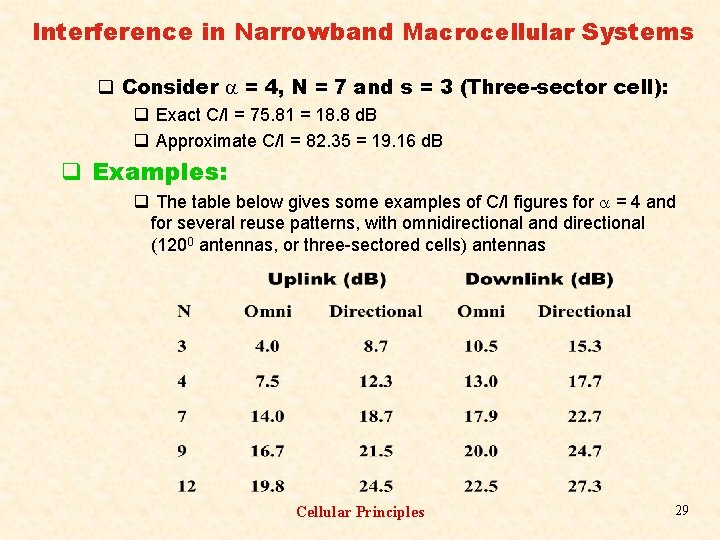Interference in Narrowband Macrocellular Systems q Consider = 4, N = 7 and s = 3 (Three-sector cell): q Exact C/I = 75. 81 = 18. 8 d. B q Approximate C/I = 82. 35 = 19. 16 d. B q Examples: q The table below gives some examples of C/I figures for = 4 and for several reuse patterns, with omnidirectional and directional (1200 antennas, or three-sectored cells) antennas Cellular Principles 29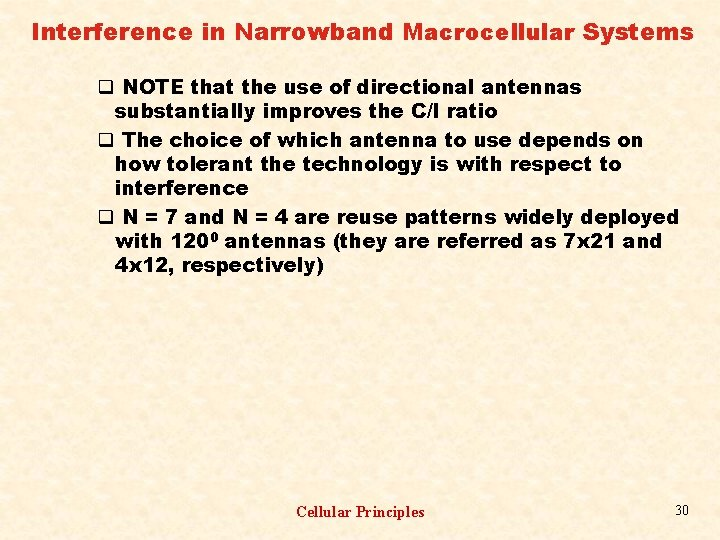Interference in Narrowband Macrocellular Systems q NOTE that the use of directional antennas substantially improves the C/I ratio q The choice of which antenna to use depends on how tolerant the technology is with respect to interference q N = 7 and N = 4 are reuse patterns widely deployed with 1200 antennas (they are referred as 7 x 21 and 4 x 12, respectively) Cellular Principles 30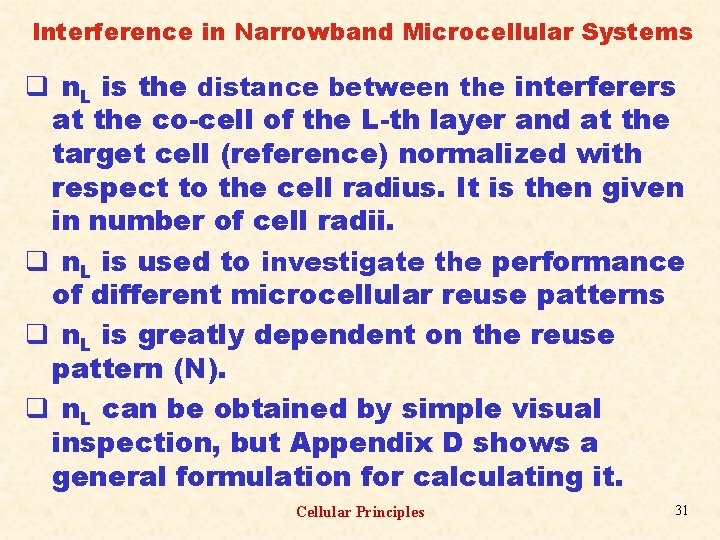Interference in Narrowband Microcellular Systems q n. L is the distance between the interferers at the co-cell of the L-th layer and at the target cell (reference) normalized with respect to the cell radius. It is then given in number of cell radii. q n. L is used to investigate the performance of different microcellular reuse patterns q n. L is greatly dependent on the reuse pattern (N). q n. L can be obtained by simple visual inspection, but Appendix D shows a general formulation for calculating it. Cellular Principles 31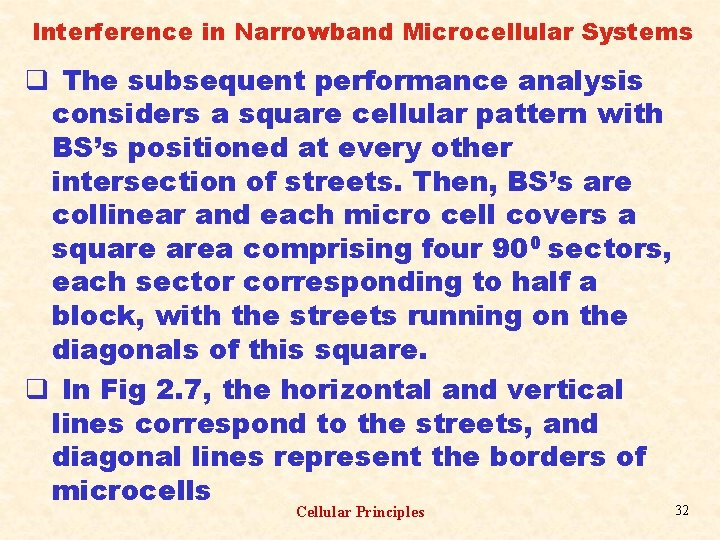Interference in Narrowband Microcellular Systems q The subsequent performance analysis considers a square cellular pattern with BS’s positioned at every other intersection of streets. Then, BS’s are collinear and each micro cell covers a square area comprising four 900 sectors, each sector corresponding to half a block, with the streets running on the diagonals of this square. q In Fig 2. 7, the horizontal and vertical lines correspond to the streets, and diagonal lines represent the borders of microcells Cellular Principles 32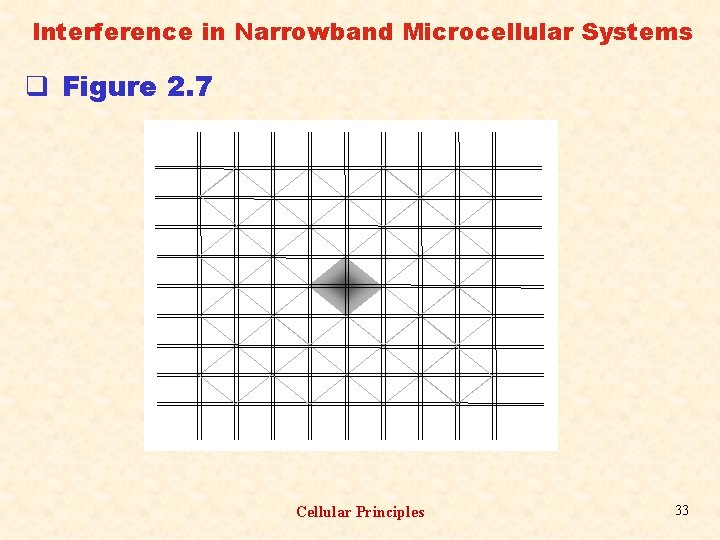Interference in Narrowband Microcellular Systems q Figure 2. 7 Cellular Principles 33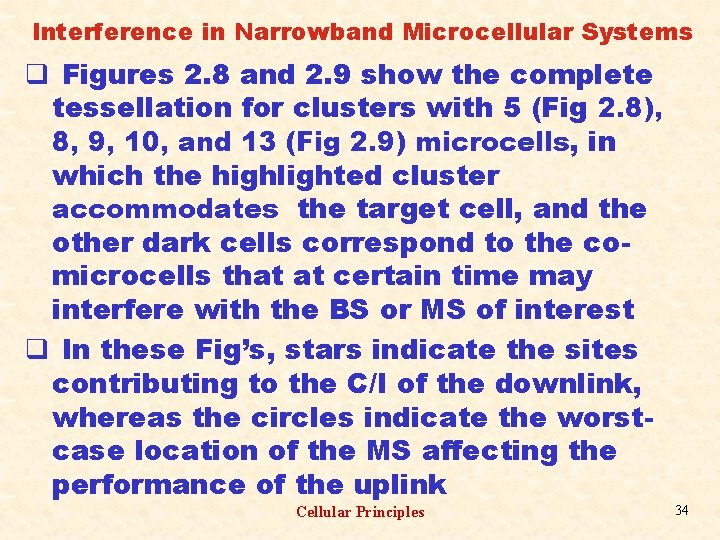Interference in Narrowband Microcellular Systems q Figures 2. 8 and 2. 9 show the complete tessellation for clusters with 5 (Fig 2. 8), 8, 9, 10, and 13 (Fig 2. 9) microcells, in which the highlighted cluster accommodates the target cell, and the other dark cells correspond to the comicrocells that at certain time may interfere with the BS or MS of interest q In these Fig’s, stars indicate the sites contributing to the C/I of the downlink, whereas the circles indicate the worstcase location of the MS affecting the performance of the uplink Cellular Principles 34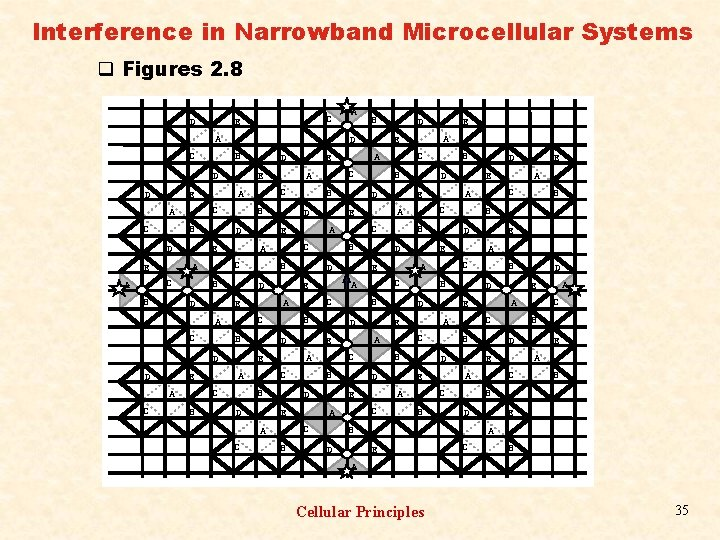Interference in Narrowband Microcellular Systems q Figures 2. 8 D C E A C B D B D D C C B D D C B C C B B D E A E C B B D A A C A B E D E C D A E D A B B E C D A E A C C B B E E C D A E C B B D A E C A B D A E C B A E C A B D A E C A B E D E C D A E D A B B E C D A E A D B B E C C D A E C B B E A E C D A E C A B D A E C A D A E B D D D A E C B A Cellular Principles 35Interference in Narrowband Microcellular Systems q Figures 2. 9 (a) Cellular Principles 36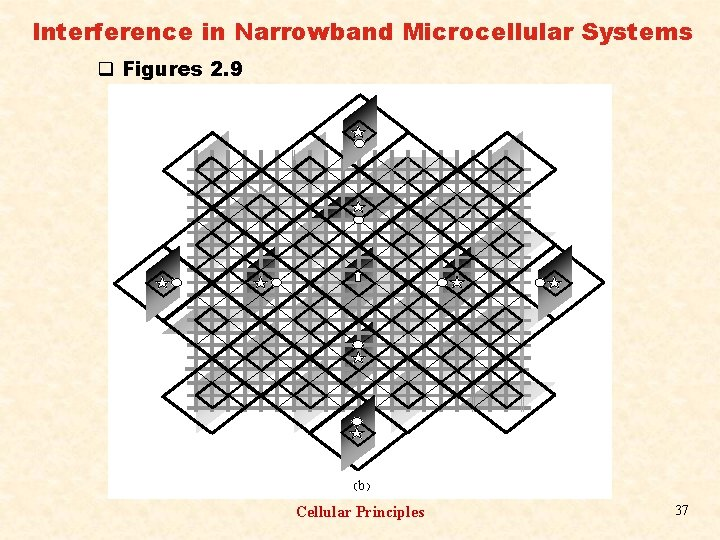Interference in Narrowband Microcellular Systems q Figures 2. 9 (b ) Cellular Principles 37Interference in Narrowband Microcellular Systems q Figures 2. 9 (c) Cellular Principles 38Interference in Narrowband Microcellular Systems q Figures 2. 9 (d) Cellular Principles 39Interference in Narrowband Microcellular Systems q Note that distinct situations can affect in different ways the performance of the downlink and the uplink q In general, the set of micro cells affecting the downlink is a subset of those influencing the uplink q Note that the staggered nature of some patterns implies that the closest interferers are either completely obstructed or obstructed for most of the time with a LOS interferer appearing many blocks away Cellular Principles 40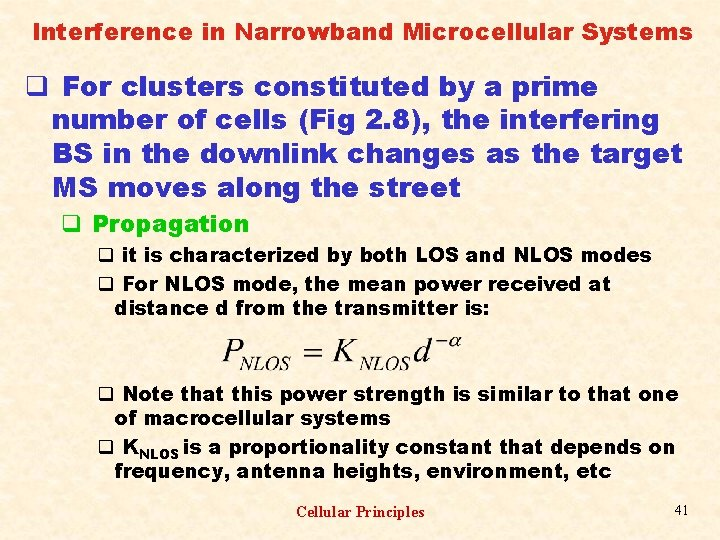Interference in Narrowband Microcellular Systems q For clusters constituted by a prime number of cells (Fig 2. 8), the interfering BS in the downlink changes as the target MS moves along the street q Propagation q it is characterized by both LOS and NLOS modes q For NLOS mode, the mean power received at distance d from the transmitter is: q Note that this power strength is similar to that one of macrocellular systems q KNLOS is a proportionality constant that depends on frequency, antenna heights, environment, etc Cellular Principles 41Interference in Narrowband Microcellular Systems q For LOS condition, and for a transmitting antenna height ht, a receiving antenna height hr, and a wavelength , the received mean power at distance d is approximately: q KLOS is a proportionality constant and depends on frequency, antenna heights, environment, etc q d. B is the breakpoint distance (4 hthr/ ) q Note that LOS and NLOS propagation modes a rather different q For NLOS condition, the mean signal strength decreases monotonically with the distance Cellular Principles 42Interference in Narrowband Microcellular Systems q For LOS condition and d < d. B, the mean signal strength decreases monotonically with a power law close to the free space condition ( 2). However, for d > d. B, the power law follows closely that of the plane earth propagation ( 4) q For calculation purposes, it is defined r = d/R as the distance of the serving BS to the MS normalized with respect to the cell radius (0 r 1), and k = R/d. B as the ratio between the cell radius and the breakpoint distance (K 0) q It is interesting to investigate the C/I performance as the mobile moves away from the serving BS along the radial street. Note: this pattern is different from the macrocellular one, whose interference pattern is approximately maintained throughout the cell Cellular Principles 43Interference in Narrowband Microcellular Systems q Uplink Interference q By using PLOS for both wanted and interfering signals: good approximation q. Downlink Interference q Following the same procedure as the uplink interference, C/I can be found. However, since this ratio greatly depends on the position of the target MS within the cell, three different interfering conditions may be identified as MS moves along the street: (1) at the vicinity of the serving BS, (2) away from both the vicinity of the serving BS and the cell border, and (3) near the cell border. Cellular Principles 44Interference in Narrowband Microcellular Systems q at the vicinity of the serving base station, more specifically at the intersection of the streets (r normalized distance from the cell site to the beginning of the block), the MS has a good radio path to its serving BS, but it also has radio paths to the interfering BS on both crossing streets. Then: q Away from the vicinity of the serving BS and away from the cell border, which correspond to most of the paths, the MS enters the block and loses LOS to those BS located on the perpendicular street. . . Cellular Principles 45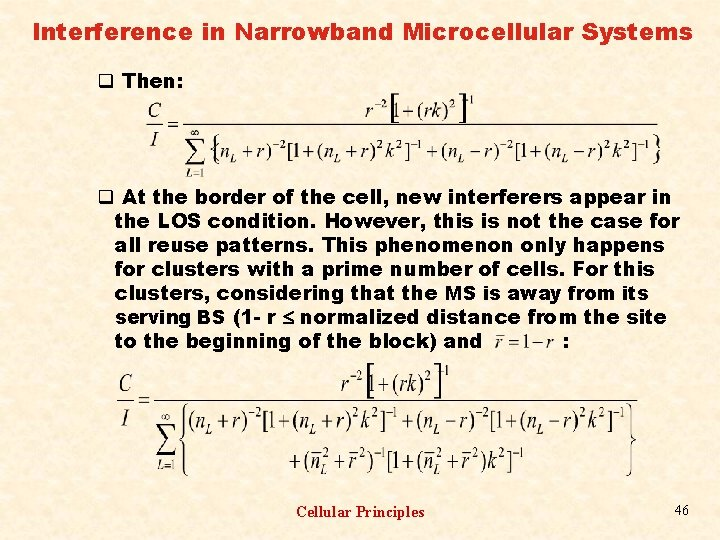Interference in Narrowband Microcellular Systems q Then: q At the border of the cell, new interferers appear in the LOS condition. However, this is not the case for all reuse patterns. This phenomenon only happens for clusters with a prime number of cells. For this clusters, considering that the MS is away from its serving BS (1 - r normalized distance from the site to the beginning of the block) and : Cellular Principles 46Interference in Narrowband Microcellular Systems q A good approximation for the downlink C/I can be obtained by simply considering L=1 q Examples q C/I performance for clusters with 5, 8, 9, 10, 13 micro cells are illustrated. The performance has been evaluated with the central micro cell as the target cell and with the MS departing from the cell center towards its edge (see arrow in Fig 2. 8, which also shows, in gray, the micro-cells that at certain time may interfere with the wanted MS in a LOS condition). q For numerical results, the calculations considered: R=100 m, street width of 15 m, ht=4 m, hr=1. 5 m, f=890 MHz ( = 3/8. 9 m), and then, K=1. 405 (note that R is 40. 5% greater than d. B). The network was considered to have an infinite number of cells (in practice, 600 layers of interfering cells) Cellular Principles 47Interference in Narrowband Microcellular Systems q Figs 2. 10 and 2. 11 show, respectively, the uplink and downlink performances for N = 5, 8, 9, 10, and 13 as a function of the normalized distance. q In general, the larger the cluster, the better the C/I. However, the five-micro-cell cluster exhibits a remarkable behavior. Its uplink C/I curve coincides with that for N=8 (lower curve in Fig 2. 10), and its downlink C/I curve coincides with that for N=10 for most of the path extension (curve below the upper curve in Fig 2. 11). In the latter, the separation of the curves occurs at the edge of the micro cell, where 2 interferers appears in a LOS condition. q Note also that in Fig 2. 10, the C/I curves for N=9 and N=13 are also coincident q Fig 2. 12 compares the performance between 5 - and 10 - micro cell clusters. Cellular Principles 48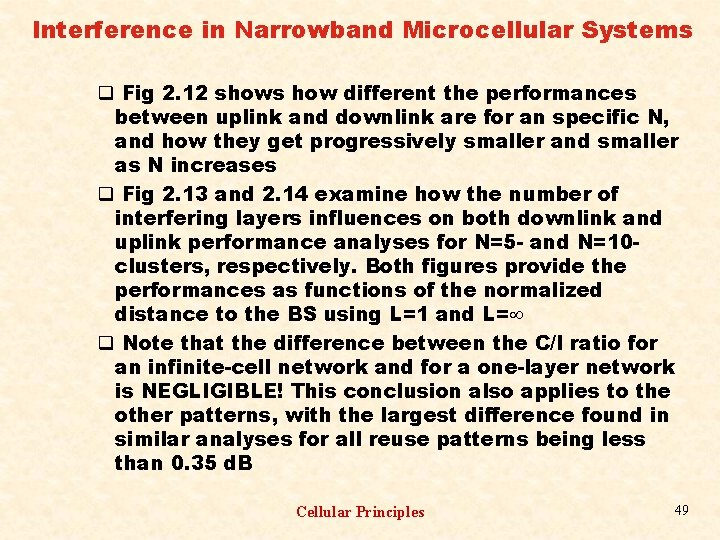Interference in Narrowband Microcellular Systems q Fig 2. 12 shows how different the performances between uplink and downlink are for an specific N, and how they get progressively smaller and smaller as N increases q Fig 2. 13 and 2. 14 examine how the number of interfering layers influences on both downlink and uplink performance analyses for N=5 - and N=10 clusters, respectively. Both figures provide the performances as functions of the normalized distance to the BS using L=1 and L= q Note that the difference between the C/I ratio for an infinite-cell network and for a one-layer network is NEGLIGIBLE! This conclusion also applies to the other patterns, with the largest difference found in similar analyses for all reuse patterns being less than 0. 35 d. B Cellular Principles 49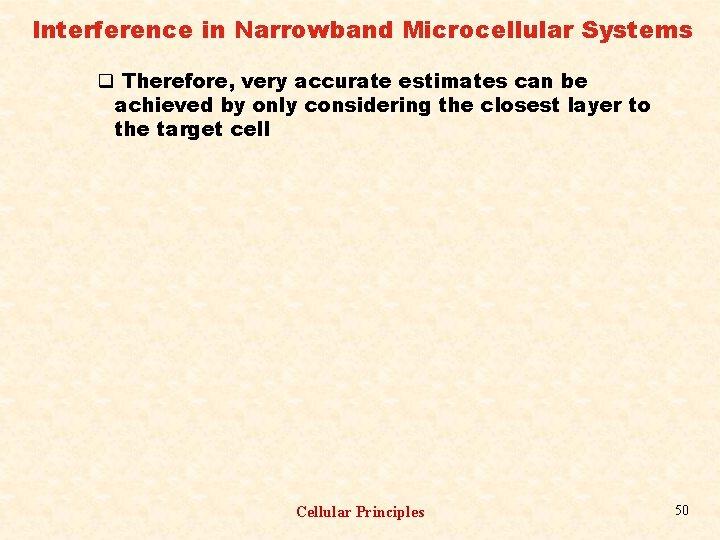Interference in Narrowband Microcellular Systems q Therefore, very accurate estimates can be achieved by only considering the closest layer to the target cell Cellular Principles 50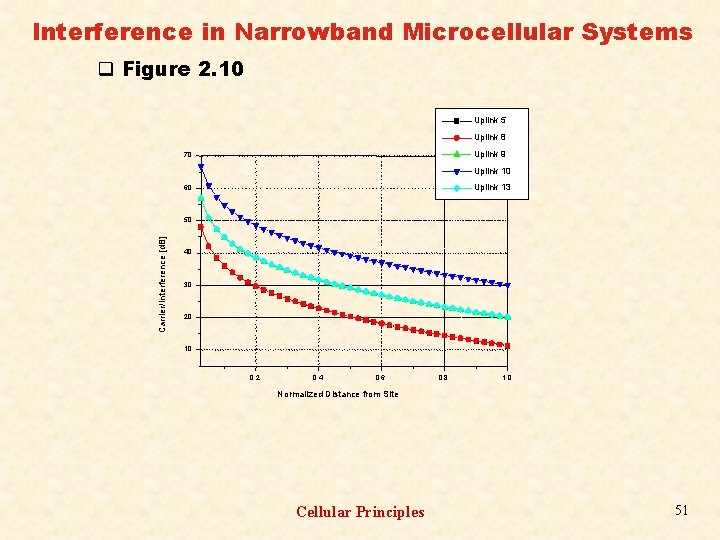Interference in Narrowband Microcellular Systems q Figure 2. 10 Uplink 5 Uplink 8 Uplink 9 70 Uplink 13 60 Carrier/Interference [d. B] 50 40 30 20 10 0, 2 0, 4 0, 6 0, 8 1, 0 Normalized Distance from Site Cellular Principles 51Interference in Narrowband Microcellular Systems q Figure 2. 11 Downlink 5 Downlink 8 Downlink 9 Downlink 10 Downlink 13 90 Carrier/Interference [d. B] 80 70 60 50 40 30 20 10 0. 2 0. 4 0. 6 0. 8 1. 0 Normalized Distance from Site Cellular Principles 52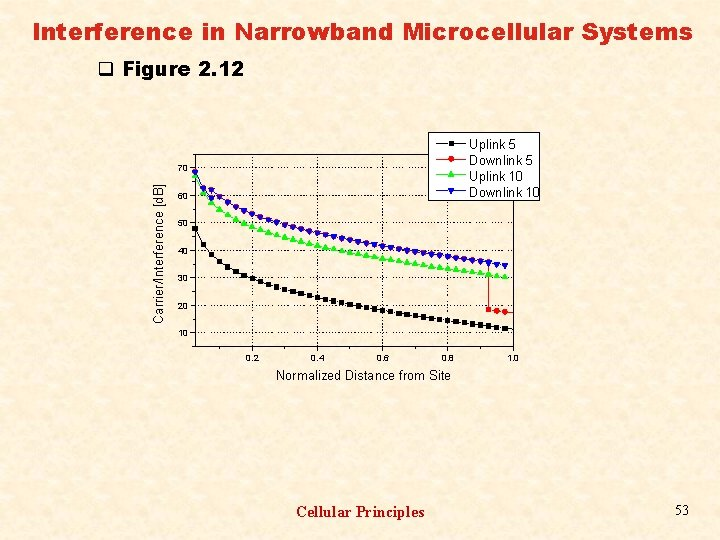Interference in Narrowband Microcellular Systems q Figure 2. 12 Uplink 5 Downlink 5 Uplink 10 Downlink 10 Carrier/Interference [d. B] 70 60 50 40 30 20 10 0. 2 0. 4 0. 6 0. 8 1. 0 Normalized Distance from Site Cellular Principles 53Interference in Narrowband Microcellular Systems q Figure 2. 13 5 Cell Clusters Uplink oo layers Uplink 1 layer Downlink oo layers Downlink 1 layer Carrier/Interference [d. B] 70 60 50 40 30 20 10 0. 2 0. 4 0. 6 0. 8 1. 0 Normalized Distance from Site Cellular Principles 54Interference in Narrowband Microcellular Systems q Figure 2. 14 8 Cell Cluster Carrier/Interference [d. B] 60 Uplink oo layers Uplink 1 layer Downlink oo layers Downlink 1 layer 50 40 30 20 10 0. 2 0. 4 0. 6 0. 8 1. 0 Normalized Distance from Site Cellular Principles 55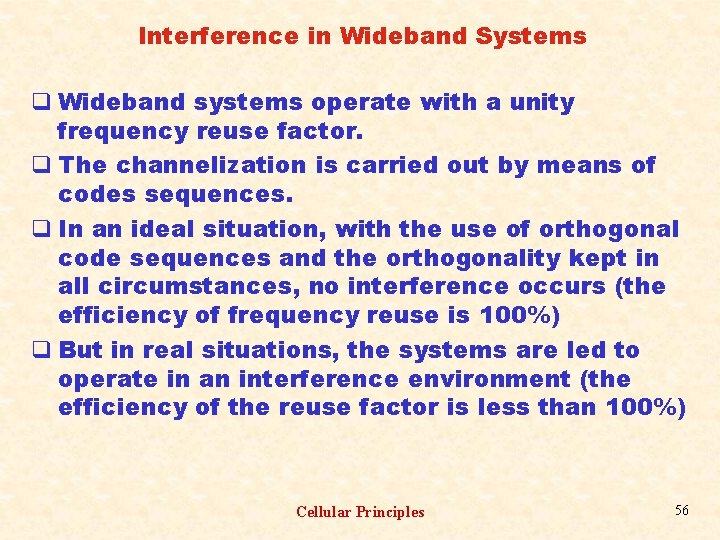Interference in Wideband Systems q Wideband systems operate with a unity frequency reuse factor. q The channelization is carried out by means of codes sequences. q In an ideal situation, with the use of orthogonal code sequences and the orthogonality kept in all circumstances, no interference occurs (the efficiency of frequency reuse is 100%) q But in real situations, the systems are led to operate in an interference environment (the efficiency of the reuse factor is less than 100%) Cellular Principles 56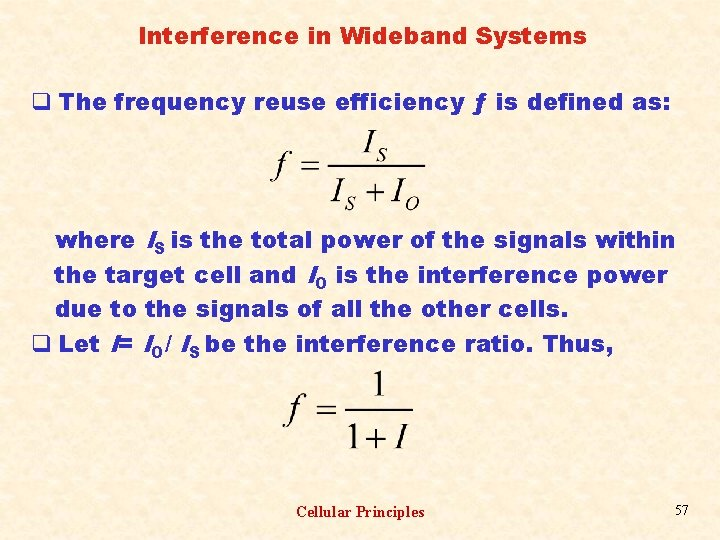Interference in Wideband Systems q The frequency reuse efficiency ƒ is defined as: where IS is the total power of the signals within the target cell and IO is the interference power due to the signals of all the other cells. q Let I= IO/ IS be the interference ratio. Thus, Cellular Principles 57Interference in Wideband Systems q Because within a system the traffic may vary from cell to cell, the frequency reuse efficiency can be defined per cell. q For an N-cell system, let j be the target cell and i the interfering cell. Therefore, for cell j, the frequency reuse efficiency, ƒj , can be written as: Cellular Principles 58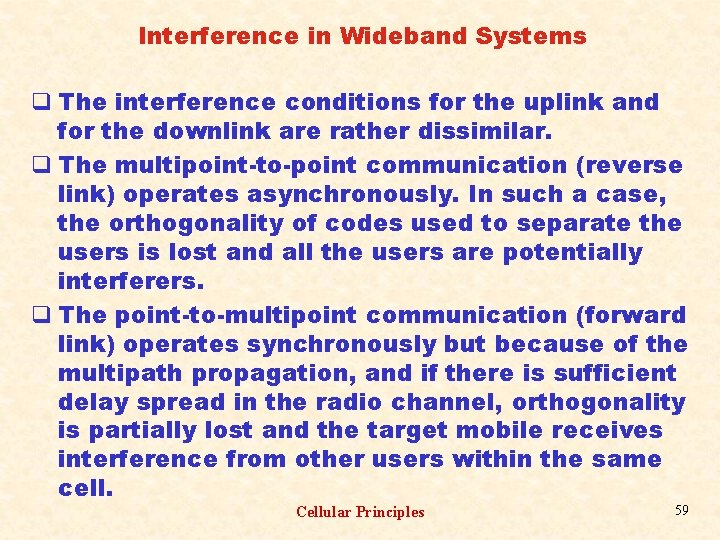Interference in Wideband Systems q The interference conditions for the uplink and for the downlink are rather dissimilar. q The multipoint-to-point communication (reverse link) operates asynchronously. In such a case, the orthogonality of codes used to separate the users is lost and all the users are potentially interferers. q The point-to-multipoint communication (forward link) operates synchronously but because of the multipath propagation, and if there is sufficient delay spread in the radio channel, orthogonality is partially lost and the target mobile receives interference from other users within the same cell. Cellular Principles 59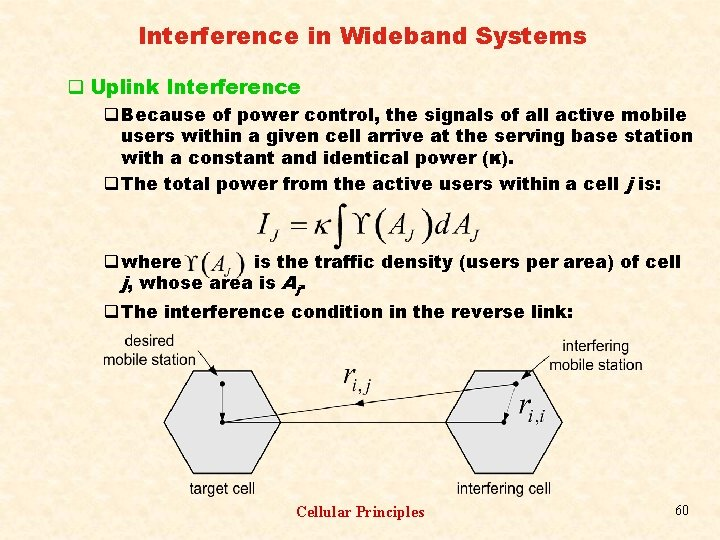Interference in Wideband Systems q Uplink Interference q Because of power control, the signals of all active mobile users within a given cell arrive at the serving base station with a constant and identical power (κ). q The total power from the active users within a cell j is: q where is the traffic density (users per area) of cell j, whose area is Aj. q The interference condition in the reverse link: Cellular Principles 60Interference in Wideband Systems q For any active user i, κ is the power at its serving base station i. q The power transmitted from the mobile station is. q The power received at the base station j (interfering power) is. q For all users in cell i the total interfering power at base station j is q Hence, Cellular Principles 61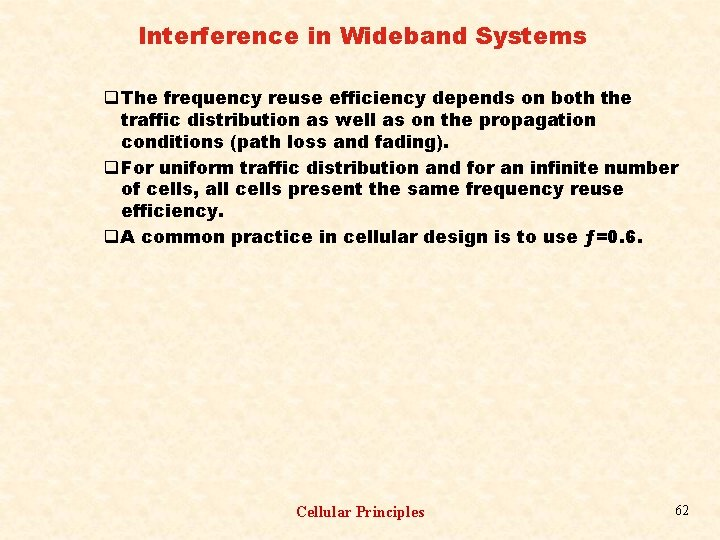Interference in Wideband Systems q The frequency reuse efficiency depends on both the traffic distribution as well as on the propagation conditions (path loss and fading). q For uniform traffic distribution and for an infinite number of cells, all cells present the same frequency reuse efficiency. q A common practice in cellular design is to use ƒ=0. 6. Cellular Principles 62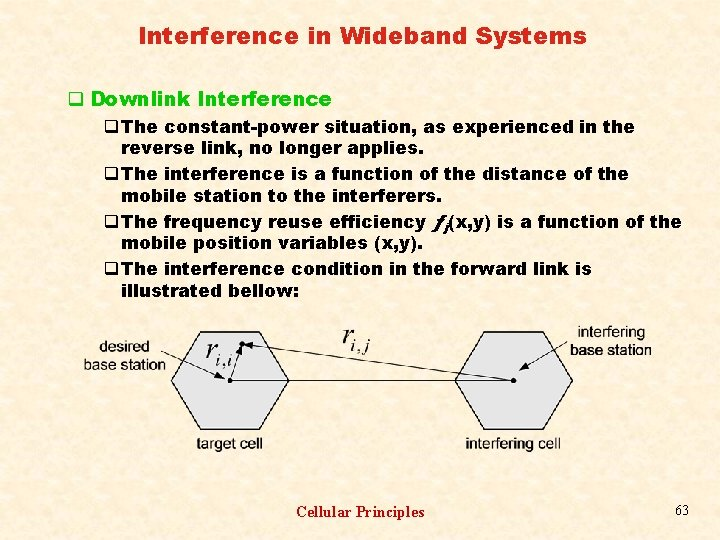Interference in Wideband Systems q Downlink Interference q The constant-power situation, as experienced in the reverse link, no longer applies. q The interference is a function of the distance of the mobile station to the interferers. q The frequency reuse efficiency ƒj(x, y) is a function of the mobile position variables (x, y). q The interference condition in the forward link is illustrated bellow: Cellular Principles 63Interference in Wideband Systems q The mean frequency reuse efficiency is defined as: q The own-cell interference at the mobile station depends on the degree of orthogonality of the codes. q For an ideal condition, no own-cell interference occurs and the frequency reuse efficiency is 1. q For a complete loss of orthogonality, the own-cell interference reaches its maximum and the reuse efficiency its minimum. q A common practice in cellular design is to use ƒ=0. 6. Cellular Principles 64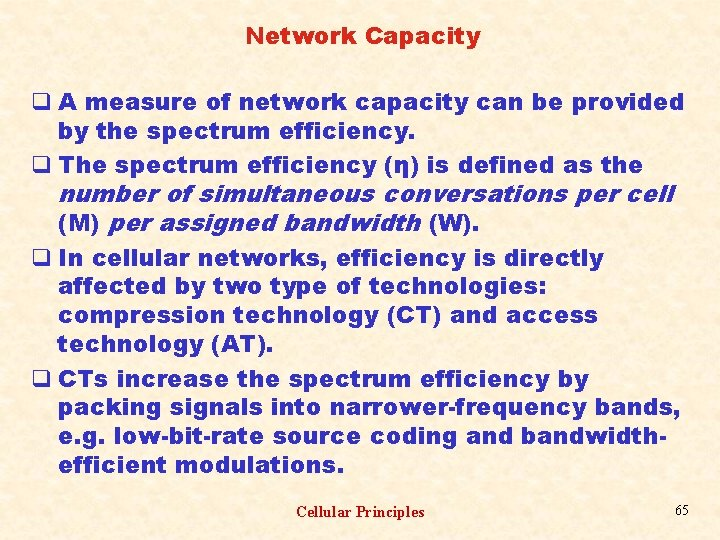Network Capacity q A measure of network capacity can be provided by the spectrum efficiency. q The spectrum efficiency (η) is defined as the number of simultaneous conversations per cell (M) per assigned bandwidth (W). q In cellular networks, efficiency is directly affected by two type of technologies: compression technology (CT) and access technology (AT). q CTs increase the spectrum efficiency by packing signals into narrower-frequency bands, e. g. low-bit-rate source coding and bandwidthefficient modulations. Cellular Principles 65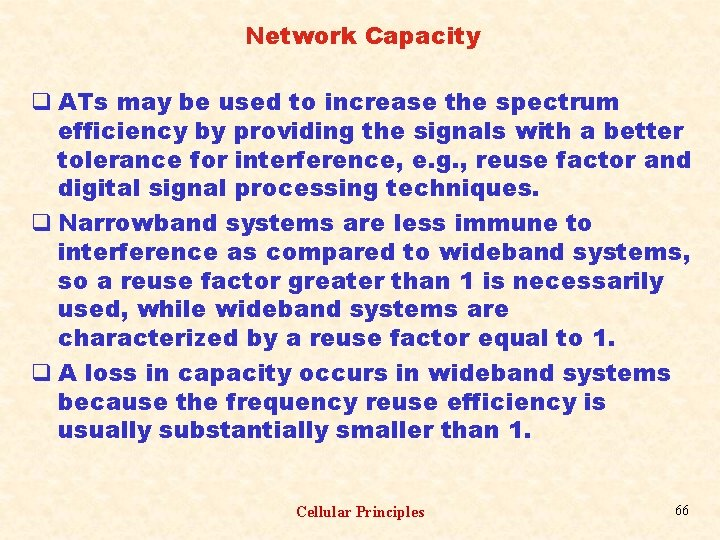Network Capacity q ATs may be used to increase the spectrum efficiency by providing the signals with a better tolerance for interference, e. g. , reuse factor and digital signal processing techniques. q Narrowband systems are less immune to interference as compared to wideband systems, so a reuse factor greater than 1 is necessarily used, while wideband systems are characterized by a reuse factor equal to 1. q A loss in capacity occurs in wideband systems because the frequency reuse efficiency is usually substantially smaller than 1. Cellular Principles 66Network Capacity q Narrowband systems are usually based on FDMA or TDMA access technologies. Wideband systems, in general, make use of CDMA access technology. q Narrowband systems q The assigned bandwidth is split into a number of subbands. The total time of each subband channel may be further split into a number of slots. q If C is the number of slots per subband times number of subbands, the spectrum efficiency is given by: Cellular Principles 67Network Capacity q The ratio C/W is a direct result of the CTs used. q The reuse factor N is chosen such that it achieves the signal-to-interference ratio required to meet transmission quality specifications. q Wideband Systems q They are typically interference limited, with the interference given by the number of active users within the system. q The total interference power It is defined as: It=IS+IO+IN, where IN is thermal noise power, IS is the power of the signals within the target cell and IO the interference power due to the signals of all the other cells. q The number of active users, their geographic distribution, and their channel activity affect the interference conditions of the systems. Cellular Principles 68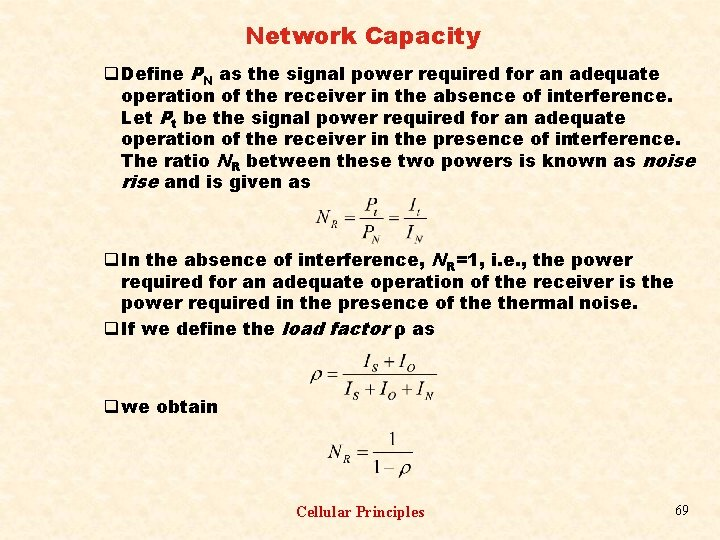Network Capacity q Define PN as the signal power required for an adequate operation of the receiver in the absence of interference. Let Pt be the signal power required for an adequate operation of the receiver in the presence of interference. The ratio NR between these two powers is known as noise rise and is given as q In the absence of interference, NR=1, i. e. , the power required for an adequate operation of the receiver is the power required in the presence of thermal noise. q If we define the load factor ρ as q we obtain Cellular Principles 69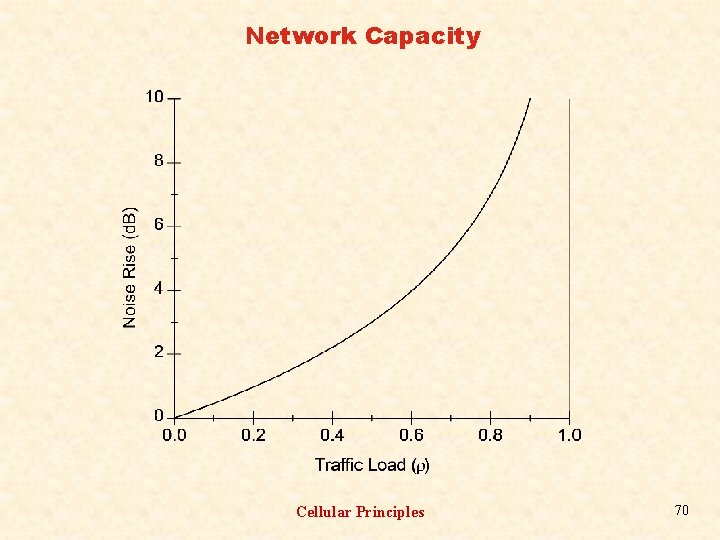Network Capacity Cellular Principles 70Network Capacity q The condition ρ=0 signifies no active users within the system. As ρ approaches unity the noise rise tends to infinity, and the system reaches its pole capacity. q A system is usually designed to operate with a loading factor smaller than 1 (typically ρ 0. 5, or equivalently 3 d. B of noise rise). q The load factor is calculated differently for the uplink and for the downlink. q Uplink Load Factor q Let i = Ei / Ni be the ratio between the energy per bit and the noise spectral density for user i. Define Gi = W / Ri as the processing gain for user i. The energy per bit is obtained as Ei = Pi Ti = Pi / Ri , where Pi , Ti and Ri = 1/ Ti are, respectively, the signal power received from user i, the bit period of user i, and the bit rate of user i. The noise spectral density is calculated as Ni = IN /W = (It – Pi ) / W. Cellular Principles 71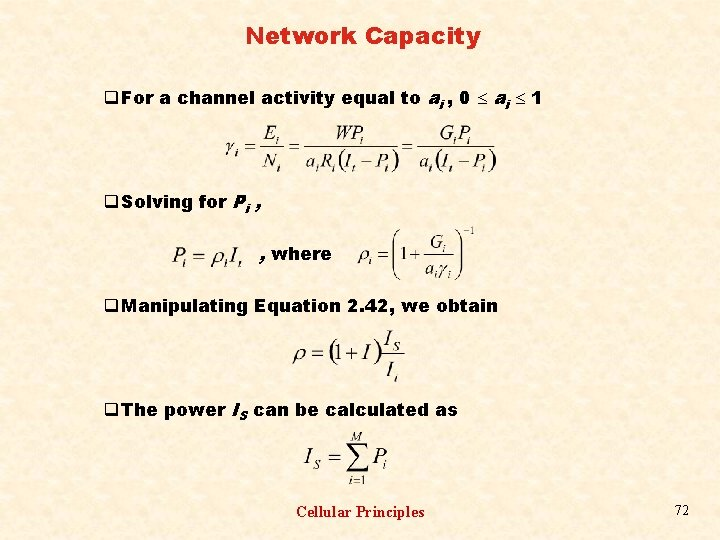Network Capacity q For a channel activity equal to ai , 0 ai 1 q Solving for Pi , , where q Manipulating Equation 2. 42, we obtain q The power IS can be calculated as Cellular Principles 72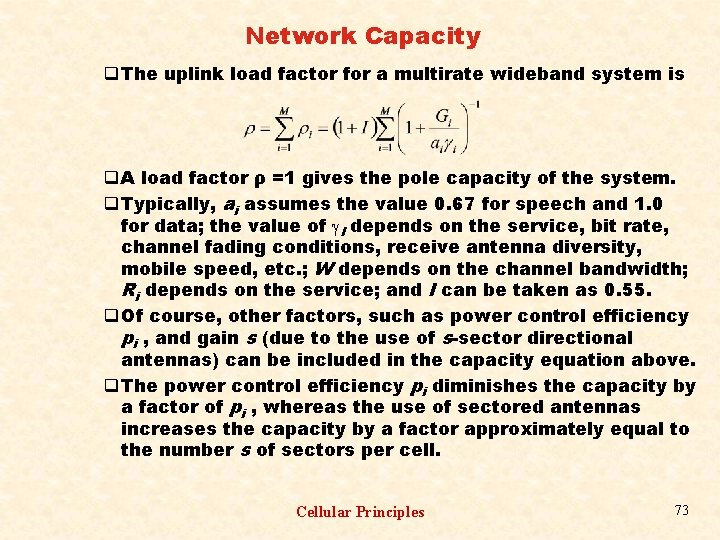Network Capacity q The uplink load factor for a multirate wideband system is q A load factor ρ =1 gives the pole capacity of the system. q Typically, ai assumes the value 0. 67 for speech and 1. 0 for data; the value of I depends on the service, bit rate, channel fading conditions, receive antenna diversity, mobile speed, etc. ; W depends on the channel bandwidth; Ri depends on the service; and I can be taken as 0. 55. q Of course, other factors, such as power control efficiency pi , and gain s (due to the use of s-sector directional antennas) can be included in the capacity equation above. q The power control efficiency pi diminishes the capacity by a factor of pi , whereas the use of sectored antennas increases the capacity by a factor approximately equal to the number s of sectors per cell. Cellular Principles 73Network Capacity q For a classical all-voice network, such as the 2 G CDMA system, all M users share the same type of constant-bitrate service, In this case q We have assumed the condition q The spectrum efficiency is q Downlink Load Factor q Because of the multipath propagation, and if there is sufficient delay spread in the radio channel, orthogonality (of the codes) is partially lost and the target mobile receives interference from other users within the same cell. Cellular Principles 74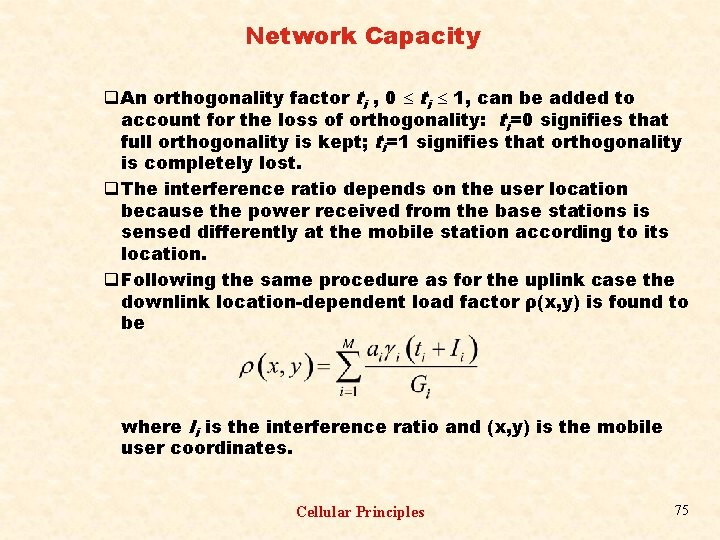Network Capacity q An orthogonality factor ti , 0 ti 1, can be added to account for the loss of orthogonality: ti=0 signifies that full orthogonality is kept; ti=1 signifies that orthogonality is completely lost. q The interference ratio depends on the user location because the power received from the base stations is sensed differently at the mobile station according to its location. q Following the same procedure as for the uplink case the downlink location-dependent load factor ρ(x, y) is found to be where Ii is the interference ratio and (x, y) is the mobile user coordinates. Cellular Principles 75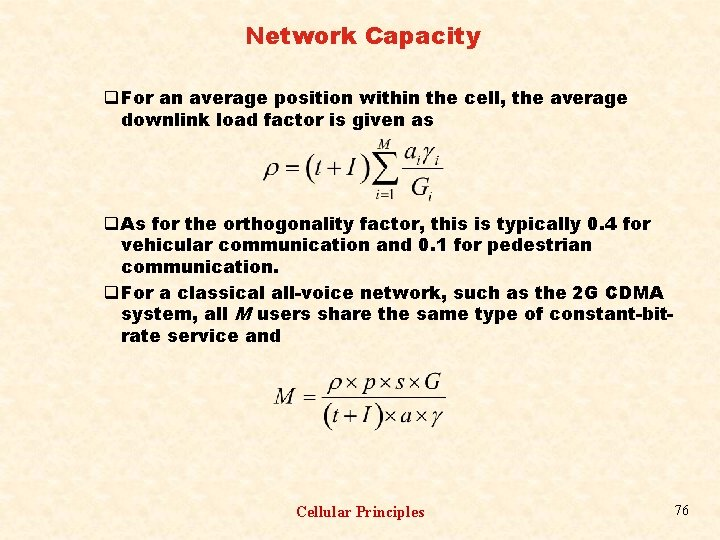Network Capacity q For an average position within the cell, the average downlink load factor is given as q As for the orthogonality factor, this is typically 0. 4 for vehicular communication and 0. 1 for pedestrian communication. q For a classical all-voice network, such as the 2 G CDMA system, all M users share the same type of constant-bitrate service and Cellular Principles 76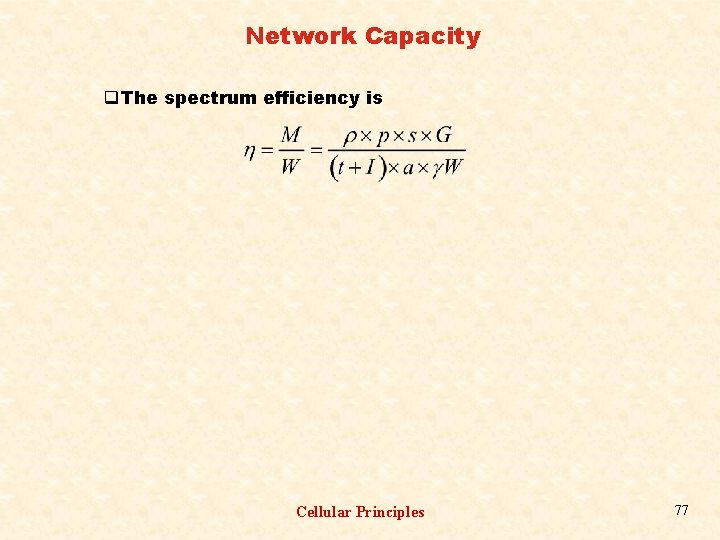Network Capacity q The spectrum efficiency is Cellular Principles 77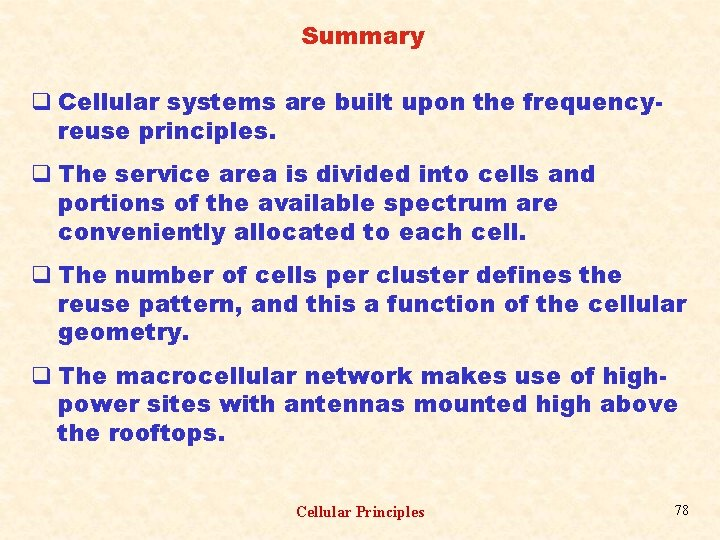Summary q Cellular systems are built upon the frequencyreuse principles. q The service area is divided into cells and portions of the available spectrum are conveniently allocated to each cell. q The number of cells per cluster defines the reuse pattern, and this a function of the cellular geometry. q The macrocellular network makes use of highpower sites with antennas mounted high above the rooftops. Cellular Principles 78Summary q The macrocellular structure serves low-capacity systems and is composed of the hexagonal cell grid. q In microcellular systems, with low power sites and antennas mounted at street level, the assumed propagation symmetry of the macrocellular network no longer applies and the hexagonal cell pattern does not make sense. q In the microcellular structure, the buildings lining each side of the street work as waveguides, in the radial direction, and as obstructors, in the perpendicular direction. Cellular Principles 79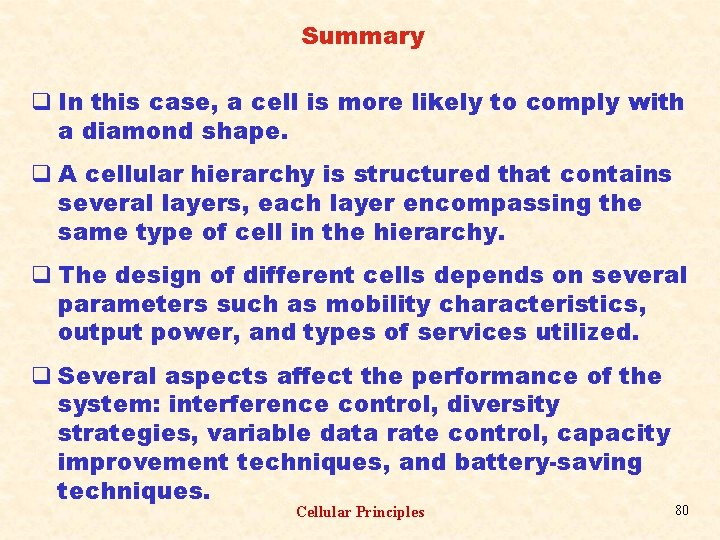Summary q In this case, a cell is more likely to comply with a diamond shape. q A cellular hierarchy is structured that contains several layers, each layer encompassing the same type of cell in the hierarchy. q The design of different cells depends on several parameters such as mobility characteristics, output power, and types of services utilized. q Several aspects affect the performance of the system: interference control, diversity strategies, variable data rate control, capacity improvement techniques, and battery-saving techniques. Cellular Principles 80Summary q Narrowband wideband systems are affected differently by interference. q In narrowband systems, interference is caused by a small number of high-power signals. Macrocellular and microcellular networks undergo different interference patterns. q In macrocellular systems, uplink and downlink present approximately the same interference performance. q In microcellular systems, the interference performance of uplink and downlink is dissimilar. Cellular Principles 81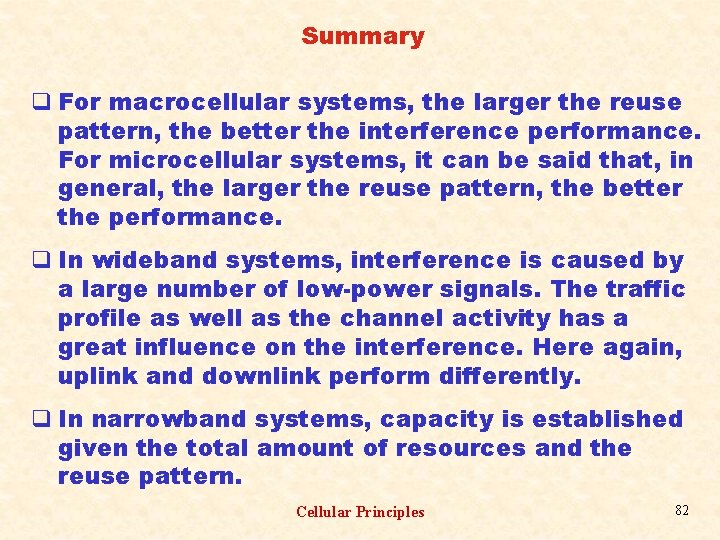Summary q For macrocellular systems, the larger the reuse pattern, the better the interference performance. For microcellular systems, it can be said that, in general, the larger the reuse pattern, the better the performance. q In wideband systems, interference is caused by a large number of low-power signals. The traffic profile as well as the channel activity has a great influence on the interference. Here again, uplink and downlink perform differently. q In narrowband systems, capacity is established given the total amount of resources and the reuse pattern. Cellular Principles 82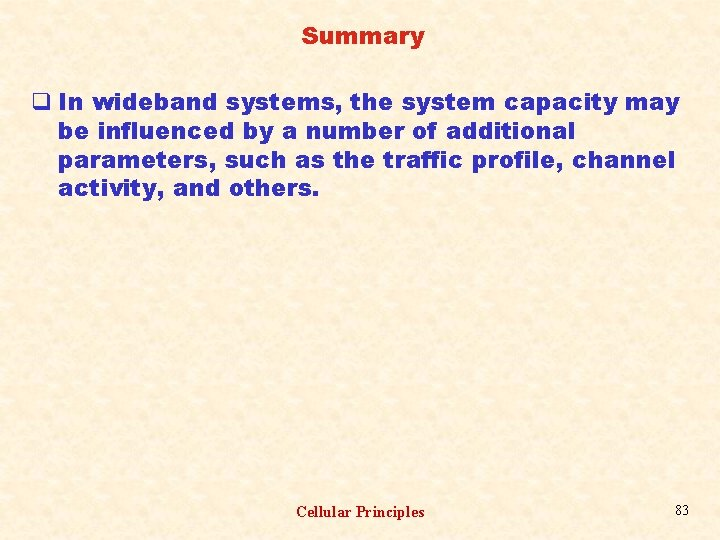Summary q In wideband systems, the system capacity may be influenced by a number of additional parameters, such as the traffic profile, channel activity, and others. Cellular Principles 83